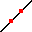Enable text based alternatives for graph display and drawing entry

Try Another Version of These Questions

Question 1
A student has a monthly budget of $100 to spend on either beers, which cost$10 each, or sodas, which cost \$5 each.

What is the largest number of beers that the student could afford to purchase in one month?

Hint

Question 2
What is the largest number of sodas the student could afford to purchase in one month?

Hint

Question 3
Draw the student's budget constraint. Put beers on the x-axis and sodas on the y-axis.

Clear All Draw:Hint 1
Hint 2

Question 4
Which combinations of beers and sodas are unaffordable?

Hint

Question 5
Indicate which combinations would leave some budget unspent.

Hint

Question 6
What is the equation for the student's budget constraint? In your equation, use Q1 as the variable to represent the quantity of beers and Q2 to represent the quantity of sodas.

Hint

Question 7
What is the opportunity cost of a beer?

sodas
Hint

Question 8
What is the opportunity cost of a soda?

beers
Hint

Question 9
How can one see the opportunity cost on the graph?

Hint